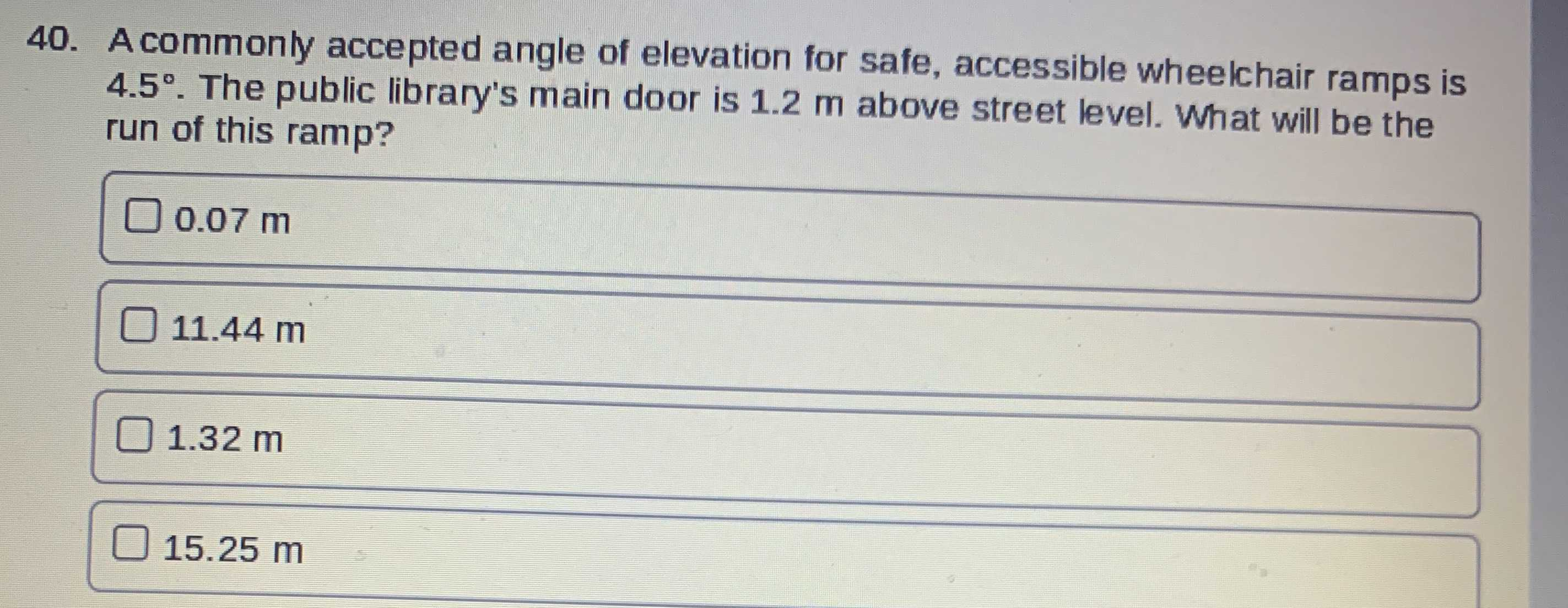### ¿Todavía tienes preguntas de matemáticas?

Pregunte a nuestros tutores expertos
Algebra
Pregunta40. A commonly accepted angle of elevation for safe, accessible wheelchair ramps is $$4.5 ^ { \circ } .$$ The public library's main door is $$1.2 m$$ above street level. What will be the run of this ramp?

$$\square 0.07 m$$

$$\square 11.44 m$$

$$\square 1.32m$$

$$\square 15.25 m$$